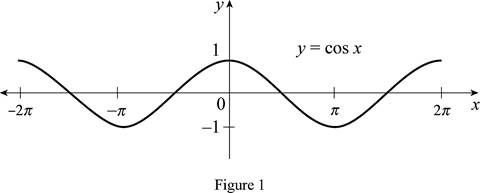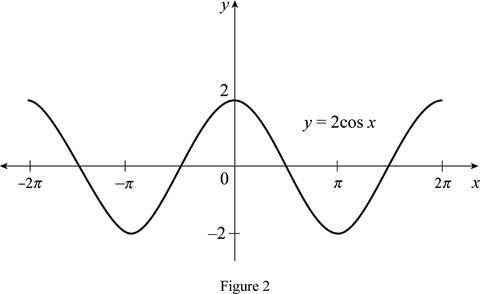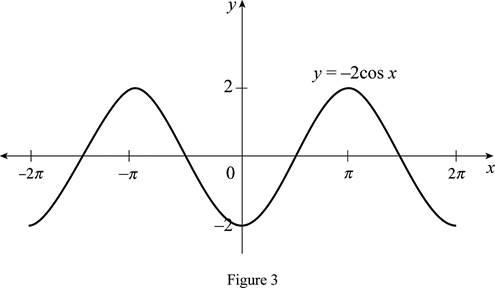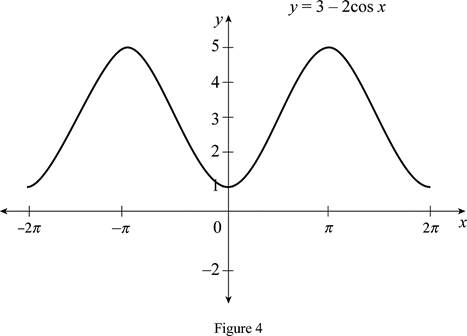# The function y = cos x by hand without plotting any points and then graph of the function y = 3 − 2 cos x .### Single Variable Calculus: Concepts...

4th Edition
James Stewart
Publisher: Cengage Learning
ISBN: 9781337687805### Single Variable Calculus: Concepts...

4th Edition
James Stewart
Publisher: Cengage Learning
ISBN: 9781337687805

#### Solutions

Chapter 1.3, Problem 18E
To determine

## To sketch: The function y=cosx by hand without plotting any points and then graph of the function y=3−2cosx .

Expert Solution

### Explanation of Solution

The standard graph of the function y=cosx is roughly drawn and shown below in Figure 1.Then, draw the graph of y=2cosx by stretching the graph of y=cosx vertically by a factor of 2. Thus, the graph of y=2cosx is shown below in Figure 2.From Figure 2, it is observed that Figure 1 is stretchedvertically by a factor of 2.

Then, draw the graph of y=2cosx by reflecting the graph of y=2cosx about the origin. Thus, the graph of y=2cosx is shown below in Figure 3.From Figure 3, it is observed that Figure 2 is reflected about the origin.

To draw the graph of y=32cosx , shift the graph y=2cosx by 3 units upward. Thus, the graph of y=32cosx is shown below in Figure 4.Observe that Figure 4is obtainedfrom Figure 1 in such a way that it is stretched vertically by a factor of 2, reflect the graph about the origin and then shift 3 units upward.

### Have a homework question?

Subscribe to bartleby learn! Ask subject matter experts 30 homework questions each month. Plus, you’ll have access to millions of step-by-step textbook answers!